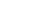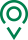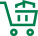• EN
• 圣康首页
• {if condition="\$categorys[\$vo]['is_menu'] == 1"}
• 关于圣康
• {if condition="\$categorys[\$vo]['is_menu'] == 1"} {if condition="\$categorys[\$vo]['is_menu'] == 1"}
• 产品展示
• {if condition="\$categorys[\$vo]['is_menu'] == 1"} {if condition="\$categorys[\$vo]['is_menu'] == 1"}
• 荣誉资质
• {if condition="\$categorys[\$vo]['is_menu'] == 1"} {if condition="\$categorys[\$vo]['is_menu'] == 1"}
• 新闻资讯
• {if condition="\$categorys[\$vo]['is_menu'] == 1"} {if condition="\$categorys[\$vo]['is_menu'] == 1"}
• 联系我们
• {if condition="\$categorys[\$vo]['is_menu'] == 1"} {if condition="\$categorys[\$vo]['is_menu'] == 1"}
• 在线留言
• {if condition="\$categorys[\$vo]['is_menu'] == 1"} {if condition="\$categorys[\$vo]['is_menu'] == 1"}
• 质量管控
• {if condition="\$categorys[\$vo]['is_menu'] == 1"} {if condition="\$categorys[\$vo]['is_menu'] == 1"}
• 源头种植
• {if condition="\$categorys[\$vo]['is_menu'] == 1"} {if condition="\$categorys[\$vo]['is_menu'] == 1"}
• 过程控制
• {if condition="\$categorys[\$vo]['is_menu'] == 1"}

### 我们的宗旨

•全球精选

一站式购物方案

•多仓直发

特有次日达配送服务

•源头直采

精选正宗好食材

•天天底价

新鲜到家购不停